Index>Robot Kit>RaspTank-Pro Smart Robot Car Kit for RPi>Lesson 13 Reading Data from MPU6050
No related product information!

# Lesson 13 Reading Data from MPU6050

In this tutorial we will learn how to read data from the MPU6050 sensor.

13.1 Components & Parts

 Components Quantity Picture Raspberry Pi 1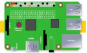Robot HAT 1MPU6050 1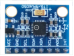Camera Module 1Camera Flex Cable 1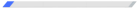13.2 Introducing MPU6050 Sensor

MPU-6050 is the world's first integrated 6-axis Motion Tracking device. It integrates a 3-axis MEMS gyroscope, a 3-axis MEMS accelerometer, and an expandable digital motion processor (DMP), and can connect a third-party digital sensor like magnetometer, with an I2C interface. After expansion, it can output a 9-axis signal through its I2C or SPI interface (SPI interface is only available in MPU-6000). MPU-60X0 can also be connected to non-inertial digital sensors (e.g., pressure sensor) through its I2C interface.

MPU-6050 uses three 16-bit ADCs (analog-to-digital converter) respectively for the gyroscope and accelerometer, and converts the measured analog quantity into an exportable digital quantity. The gyroscope can measure angular velocity, and the accelerometer do acceleration. To accurately track fast and slow motion, the measuring range of the sensor is adjustable: range of the gyroscope is ±250, ±500, ±1000, ±2000°/sec (dps, or degrees per second), and that of the accelerometer is ±2, ±4, ±8, ±16g (gravitational acceleration).

An on-chip 1024-byte FIFO (first in first out) helps reduce system power consumption. A 400kHz I2C interface is used for the communication with all device registers. In addition, a temperature sensor, an oscillator with a ±1% variation in the working environment, and a programmable low-pass filter (LPF) are embedded on the chip.

As for power, the MPU-6050 module supports a VCC's voltage operation range of 3-5VDC.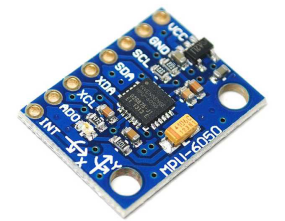13.3 Schematic Diagram (Wiring)

Connect the MPU6050 module to the MPU6050 port on the Robot HAT, as shown below: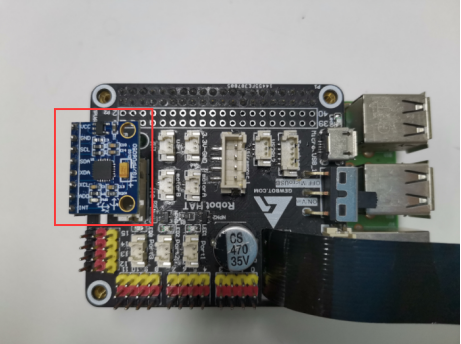The positive direction of the X, Y, and Z axes are shown as the arrows in the following picture: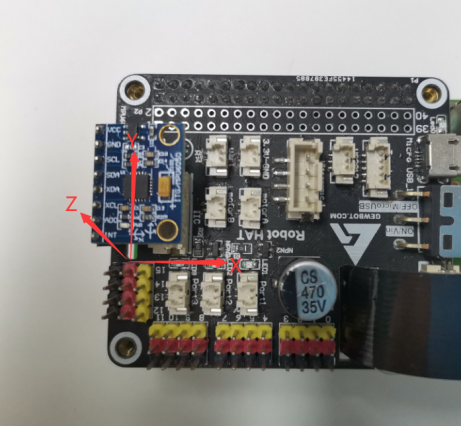### Running the Code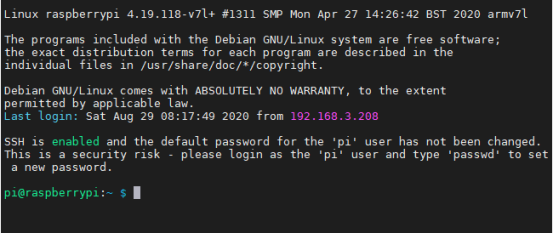2. Type in the command below and press Enter to go to the folder of the program: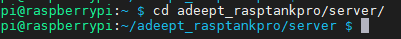3. View the current content in the folder:

ls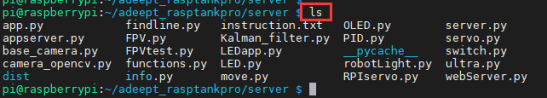4. Type in the command below and press Enter to run the program:

sudo python3 mpu6050Test.py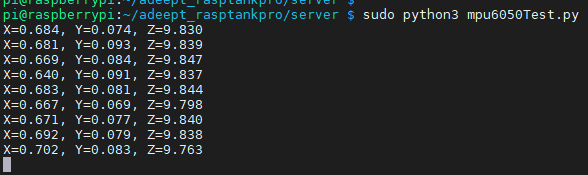5. After successfully running the code, you can see the values of gravitational acceleration at X, Y, and Z axes.

If the value of X is positive, it means that the mpu6050 X axis is offset upward.

If the value of X is negative, it means that the mpu6050 X axis is offset downward.

When the X value is 0, it means that the x-axis direction of mpu6050 is horizontal. When the X value is the gravitational acceleration value (approximately 9.8), it means that the x-axis direction of mpu6050 is up and perpendicular to the ground. When the X value is -9.8, Indicates that the x-axis direction of mpu6050 is in a downward vertical state. The X axis and Y axis are similar.

If the value of Y is positive, it means the mpu6050 Y axis offset upward.

If the value of Y is negative, it means the mpu6050 Y axis offset downward.

When the mpu6050 lies level (as shown above), the value of Z is equal to the gravitational acceleration (about 9.8)

If the Z-axis value is positive, the Z-axis direction of mpu6050 is upward.

If the Z-axis value is negative, the Z-axis direction of mpu6050 is down.

Minor data deviation may happen.

6. Press Ctrl + C to end the program that is running.

13.5 Main Program

Refer to the mpu6050Test.py file for more details.

 1. from mpu6050 import mpu6050  2. import time  3.   4. sensor = mpu6050(0x68)  5. def mpu6050test():  6.   x = 0  7.   y = 0  8.   z = 0  9.   for i in range(0,10):  10.     accelerometer_data = sensor.get_accel_data()  11.     x = x + accelerometer_data['x']  12.     y = y + accelerometer_data['y']  13.     z = z + accelerometer_data['z']  14.   print('X=%.3f, Y=%.3f, Z=%.3f'%(x/10.0,y/10.0,z/10.0))  15.   time.sleep(0.3)  16.   17. if __name__ == "__main__":  18.   try:  19.     while True:  20.       mpu6050test()  21.   except:  22.     pass

The function reads the initial data of MPU6050's 6 axes and accumulate them. After the loop is completed, obtain the average value to reduce deviation.